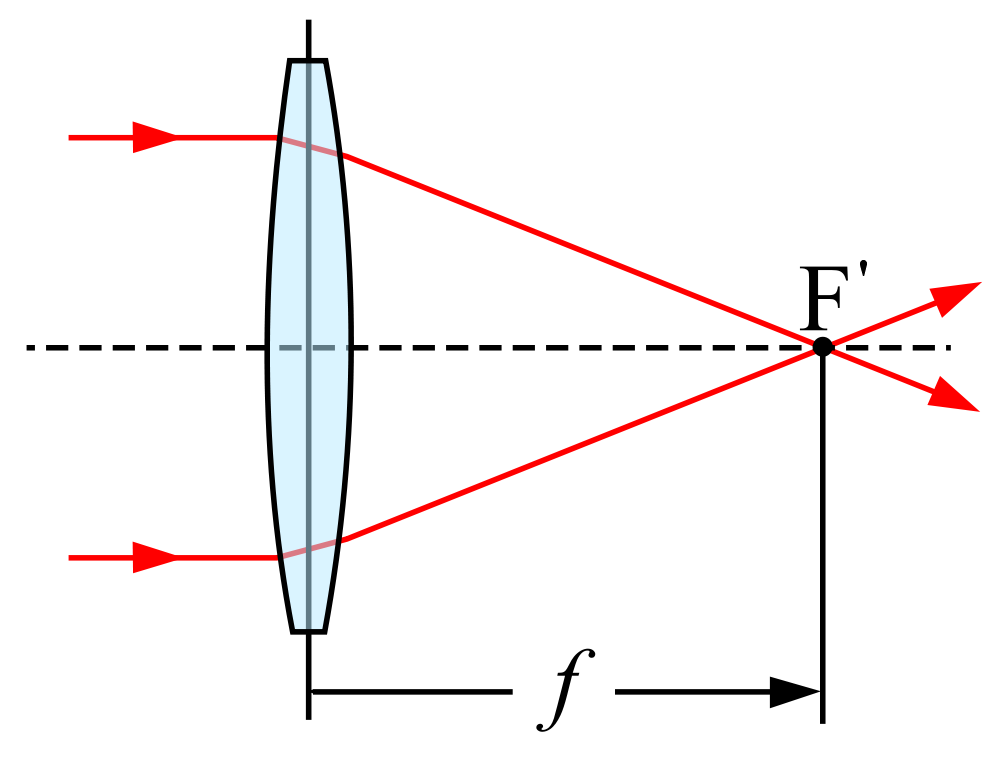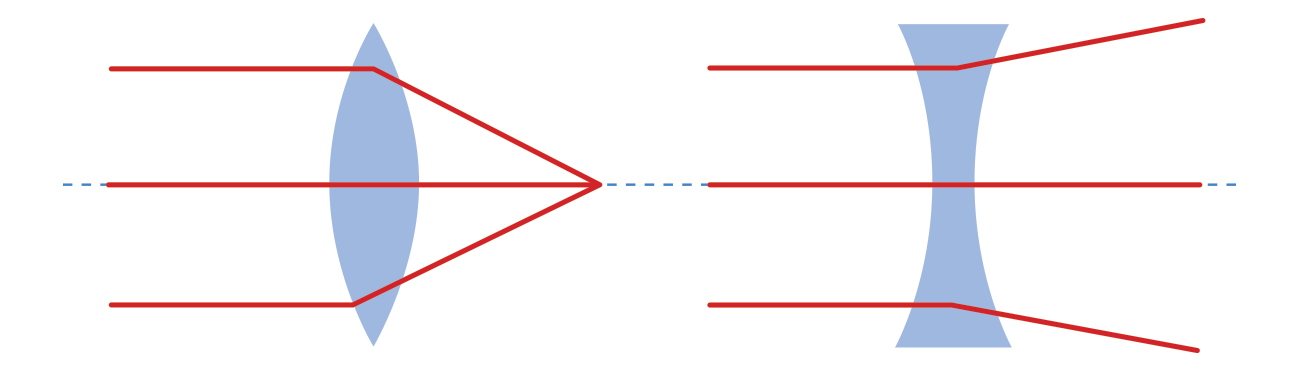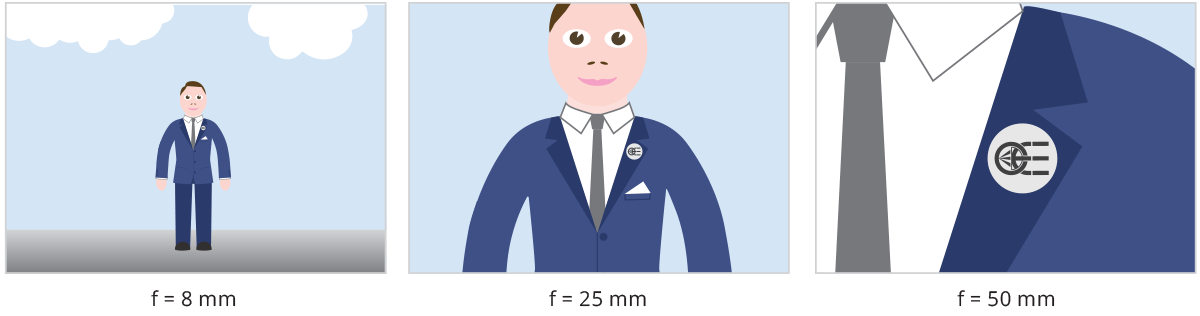## Focal length

For common optical systems, in thin lens approximation, the focal length is the distance over which collimated rays coming from infinity converge to a point on the optical axis.The focal length is a typical characteristic of an optical system. It is a measure of how strongly the system converges or diverges rays of light. If collimated rays converge to a physical point, the lens is said to be positive (convex), whereas if rays diverge the focus point is virtual and the lens is said to be negative (concave). All optics used in machine vision applications are overall positive, i.e. they focus incoming light onto the sensor plane. CCTV lenses are commonly identified by their focal length, expressed in millimeters (12mm, 25mm, 35mm, etc.).For optical systems used in machine vision, in which rays reflected from a faraway object are focused onto the sensor plane, the focal length can be also seen as a measure of how much area is imaged on the sensor (Field of View): the longer the focal length, the smaller the FoV and vice versa (this is not completely true for some particular optical systems, e.g. in astronomy and microscopy).The focal length and the focus plane coincide only when the object is placed at an infinite distance, indeed beams from a point on the object can be considered as parallel. When instead the distance from the object is ‘short’ (rule of thumb: <10x Focal length), we are in macro mode and the focus plane is placed further away from the optical system compared to the focal length.Next →